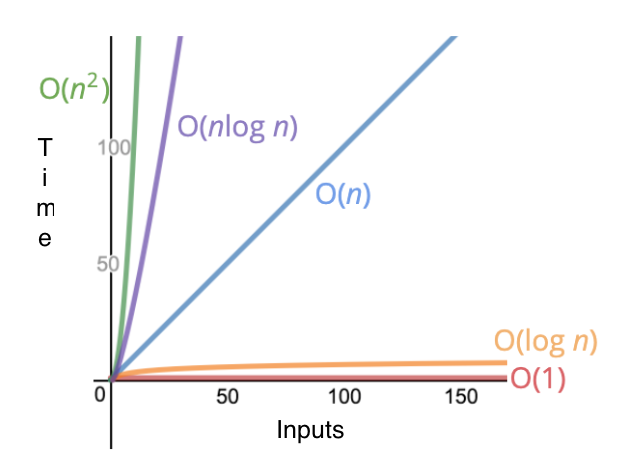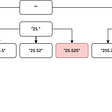# Big O Notation

## Overview of Time Complexity

As Software Engineers, our days involve writing code to solve problems. There are many ways to solve a given problem, but how are we able to tell which method is best? Is it how fast the computer runs the solution? Is it the amount of computer memory used? The answer to these questions and how our solutions are compared is through Big O notion. This blog will focus on the first question of speed, which falls under the category of Time Complexity.

One thing to clear up before diving any further into Big O notation is the concept of algorithms. An algorithm is defined as, “a finite sequence of well-defined, computer-implementable instructions, typically to solve a class of problems or to perform a computation ” (Wikipedia). Or more simply put, an algorithm is the solution to a problem and we use Big O notation to compare and discuss our algorithms.

What is Big O notation?

As mentioned previously, Big O notion provides Software Engineers a way to discuss algorithms and how they perform. To tell how fast an algorithm is, we don’t count the number of seconds it takes to run our code but instead count the number of operations the computer has to run. Or, more precisely, we measure “…how the runtime of an algorithm grows as the inputs grow” (Steele).

What does Big O look like and how do we use it?

In Big O notion, instead of counting each individual operation, we look at the algorithm’s overall trend. Moreover, as stated above, what we really want to know is how the runtime is changing as inputs increase. Algorithms typically fall in one of the these 5 Big O time complexities ranging from most to least efficient.

1. O(1)
2. O(log n)
3. O(n)
4. O(n * log n)
5. O(n^2)Visual Representation of Big O Notation Time Complexities from Colt Steele’s JavaScript Algorithms and Data Structures Masterclass Udemy Course

It’s important to note that when discussing Big O notation, we are discussing the worst case scenario of an algorithm. Looking at the worst case scenario of an algorithm is useful because it lets us see the impact of our algorithm at its extremes. For example, say we have the below algorithm that searches to see if a letter is in a given string.

`function findLetter(string, letter) {   let match = false   for (let i = 0; i < string.length; i++) {     if(string[i] === letter) {       match = true     }   }   return match}`

If the string variable we were using were “algorithms are fun” and the letter we were searching for was “n” our algorithm would have to look through every single letter before returning true. In contrast, the best case scenario would be if we were searching for the letter“c”. Since our worst case scenario is that we need to check every letter in the string once, we can say that our function has an O(n); pronounced O of n. This means that our runtime directly correlates with the number of inputs and as n or the number of letters in the string increases, so does the runtime in a linear fashion.

Next, we will look at the different types of Big O notation complexities and provide an example algorithm for each. All but one of the provided examples comes from Colt Steele’s JavaScript Algorithms and Data Structures Masterclass Udemy course, which I highly recommend.

O(1)

When an algorithm has an O(1) time complexity, the algorithm is said to have a constant run time. This means that no matter how large or small the input, the runtime will remain constant. This is the most efficient type of algorithm.

`Function that calculates the sum of all numbers from 1 to the number n.function addUpTo(n) {   return n * (n + 1) / 2;}`

In the above function, no matter what number we use for n the function will always complete the same 3 simple operations; addition, multiplication, and division.

O(log n)

Although constant run time is the most efficient type of algorithm time complexity O(log n), also known as log time, is a close second. The main characteristic of an algorithm with O(log n) is that the, “…choice of the next element on which to perform some action is one of several possibilities, and only one will need to be chosen” (Stack Overflow). A common example to represent O(log n) is a Binary Search. The Flatiron School provides an excellent example of a Binary Search as seen below.

`function findLetter(string, letter) {    let startpoint = 0    let endpoint = string.length - 1;    let guessPosition = parseInt((endpoint - startpoint)/2)while (startpoint != endpoint) {      console.log(`start point is \${startpoint}, endpoint is     \${endpoint} and guessposition is \${guessPosition}`)        if (string[guessPosition] < letter) {          console.log('too low')            startpoint = guessPosition            guessPosition = startpoint + Math.round((endpoint - startpoint)/2)        } else if(string[guessPosition] > letter) {          console.log('too high')            endpoint = guessPosition            guessPosition = startpoint + parseInt((endpoint - startpoint)/2)        } else {          console.log('just right')            return true;        }    }    if(string === letter){      return true    } else{      console.log('sorry')      return false;    }  }let string1 = "aabeeeeeeffhhiiiimmooorsssssstttttttwww"let letter1 = "t"findLetter(string1,letter1)Output: start point is 0, endpoint is 38 and guessposition is 19too lowstart point is 19, endpoint is 38 and guessposition is 29just righttrue`

What makes the Binary search so efficient is that with each iteration of the loop, the dataset is split in half until either the answer is found or not. A Binary Search is most commonly compared to how one might look up a phone number in a directory. Instead of flipping through pages one by one, the searcher will flip open a random page then check to see how close their guess was to the page they need. Although the number of guesses the searcher might need to land on the correct page increases with the number of pages in the directory, it does not directly correlate in a 1:1 fashion; it increases much more slowly (see chart below). Moreover, this is what we mean when our binary search and algorithms have an O(log n) time complexity.

O(n)

The next most efficient time complexity is O(n), which we saw in our earlier example of finding a letter in a string (see below). The run time of an algorithm with O(n) has linear runtime. This means that the number of operations grows roughly proportionally with n. Refer back to the earlier explanation of how this algorithm works and how the runtime correlates in a linear fashion with the number of inputs.

`function findLetter(string, letter) {   let match = false   for (let i = 0; i < string.length; i++) {     if(string[i] === letter) {       match = true     }   }   return match}`

O(n log n)

Although algorithms with a time complexity of O(n log n) are less efficient than the complexities we’ve discussed so far, they can have a significantly better runtime than an algorithm with O(n²) for large arrays. A common example of an algorithm with a time complexity of O(n log n) is a quicksort, which like the name suggests is a fast sorting algorithm.

`function pivot(arr, start = 0, end = arr.length - 1) {  const swap = (arr, idx1, idx2) => {    [arr[idx1], arr[idx2]] = [arr[idx2], arr[idx1]];  };  // We are assuming the pivot is always the first element  let pivot = arr[start];  let swapIdx = start;  for (let i = start + 1; i <= end; i++) {    if (pivot > arr[i]) {      swapIdx++;      swap(arr, swapIdx, i);    }  }  // Swap the pivot from the start the swapPoint  swap(arr, start, swapIdx);  return swapIdx;}function quickSort(arr, left = 0, right = arr.length -1){    if(left < right){        let pivotIndex = pivot(arr, left, right) //3        //left        quickSort(arr,left,pivotIndex-1);        //right        quickSort(arr,pivotIndex+1,right);      }     return arr;}            quickSort([100,-3,2,4,6,9,1,2,5,3,23])// Shows how the algorithm works toward a solution// [4,6,9,1,2,5,3]// [3,2,1,4,6,9,5]//        4//  3,2,1    6,9,5//      3      6//  2,1      5  9//    2//  1Output:[  -3, 1, 2, 2,  3, 4, 5, 6, 9, 23, 100]`

The way a quicksort works is that it picks an element, typically referred to as a pivot, and finds the correct index of where the element should be after the array is sorted. Then when the pivot is in the correct place, the algorithm sorts the numbers on either side of the pivot.

O(n²)

The last algorithm we will discuss is O(n²) also known as quadratic runtime. Although there are algorithms slower than O(n²), a quadratic time complexity is typically the worst complexity we are willing to accept for an algorithm.

`// function prints all the number pairs from 0 up to but not including nfunction printAllPairs(n) {  for (let i = 0; i < n; i++) {    for (let j = 0; j < n; j++) {      console.log(i, j);    }  }} printAllPairs(3)Output:0 00 10 21 01 11 22 02 12 2`

The function above has two loops, one of which is nested, or said another way; the second loop is inside of the first loop. When there is a nested loop, it is normally a clue that our algorithm has at least an O(n²) time complexity. On their own, each loop has a time complexity of O(n), meaning that the runtime increases in a linear fashion with increasing inputs. However, since the second loop is nested, this means that as n increases, the number of outputs is squared. As you can see above, when we have an input of 3, we get an output of 9 pairs or 3².

Thank you for taking the time to learn more about Big O notion and examples of different Time Complexities. Be on the lookout for the next blog, where we discuss Big O notion and Space Complexity.

Resources

Steele, C. (n.d.). JavaScript Algorithms and Data Structures Masterclass. Online Course.

Big O Approximation. (n.d.). Retrieved October 10, 2020, from https://learn.co/lessons/big-o-approximation

Feminella, J. (n.d.). What does O(log n) mean exactly? Retrieved from https://stackoverflow.com/questions/2307283/what-does-olog-n-mean-exactly?page=1

Algorithm. (2020, October 07). Retrieved October 10, 2020, from https://en.wikipedia.org/wiki/Algorithm

--

--

--

## More from The Startup

Get smarter at building your thing. Follow to join The Startup’s +8 million monthly readers & +756K followers.

## Space Shooter 2D — Project Setup## 6 Surprising Reasons IT is Changing## HyperFyre v5.0.3 Release## What happens behind the scenes when we import a module in Python## Introduction to TornadoFX Part 2## Cheat Turn Texas Holdem Poker## Compiling all project blueprints in Unreal Engine## I Wish I’d Known My Mother Couldn’t Be Trusted When I Was Young My mother doesn’t know## Matthew Sedlacek

Software Engineer — Full Stack, JavaScript, ReactJS, Ruby on Rails, OO Programming (https://www.matthewsedlacek.com/)

## TDD in-depth — insights from an Ensemble programming workshop## My Attempt at Explaining Decorator and Facade pattern## LeetCode 93 : Restore IP address — medium